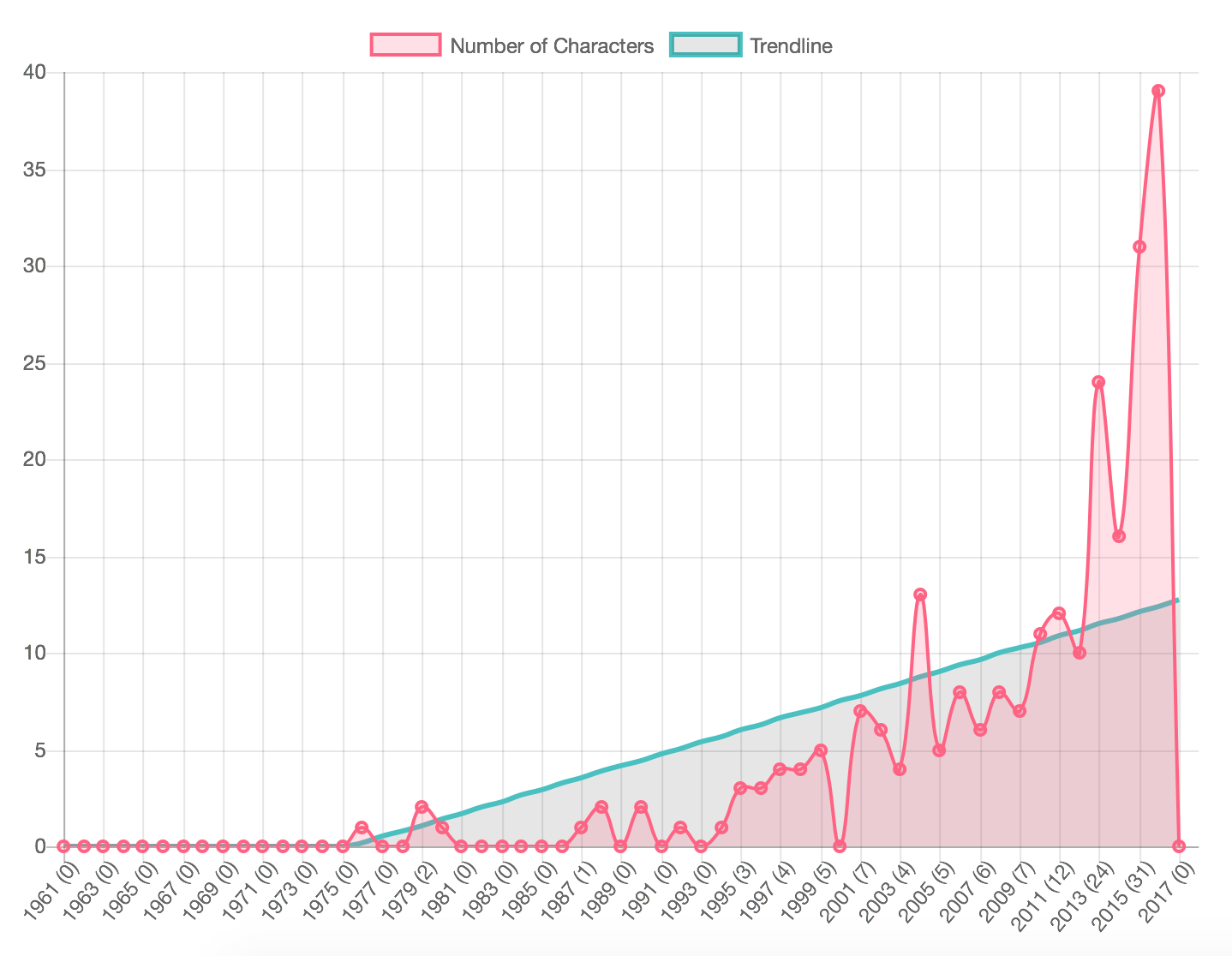Categories

# Linear Regressions in PHP

Using PHP to calculate trendiness

Sometimes math exists to give me a headache.

In calculating the deaths of queer females per year, my wife wondered what the trend was, other than “Holy sweat socks, it’s going up!” That’s called a ‘trendline’ which is really just a linear regression. I knew I needed a simple linear regression model and I knew what the formula was. Multiple the slope by the X axis value, and add the intercept (which is often a negative number), and you will calculate the points needed.

Using Google Docs to generate a trend line is easy. Enter the data and tell it to make a trend line. Using PHP to do this is a bit messier. I use Chart.js to generate my stats into pretty graphs, and while it gives me a lot of flexibility, it does not make the math easy.

I have an array of data for the years and the number of death per year. That’s the easy stuff. As of version 2.0 of Chart.js, you can stack charts, which lets me run two lines on top of each other like this:

```var myChart = new Chart(ctx, {
type: 'bar',
data: {
labels: ['Item 1', 'Item 2', 'Item 3'],
datasets: [
{
type: 'line',
label: 'Line Number One',
data: [10, 20, 30],
},
{
type: 'line',
label: 'Line Number Two',
data: [30, 20, 10],
}
]
}
});
```

But. Having the data doesn’t mean I know how to properly generate the trend. What I needed was the most basic formula solved: `y = x(slope) + intercept` and little more. Generating the slope an intercept are the annoying part.

For example, slope is `(NΣXY - (ΣX)(ΣY)) / (NΣX2 - (ΣX)2)` where,

• x and y are the variables.
• b = The slope of the regression line
• a = The intercept point of the regression line and the y axis.
• N = Number of values or elements
• X = First Score
• Y = Second Score
• ΣXY = Sum of the product of first and Second Scores
• ΣX = Sum of First Scores
• ΣY = Sum of Second Scores
• ΣX2 = Sum of square First Scores

If that made your head hurt, here’s the PHP to calculate it (thanks to Richard Thome ):

```	function linear_regression( \$x, \$y ) {

\$n     = count(\$x);     // number of items in the array
\$x_sum = array_sum(\$x); // sum of all X values
\$y_sum = array_sum(\$y); // sum of all Y values

\$xx_sum = 0;
\$xy_sum = 0;

for(\$i = 0; \$i < \$n; \$i++) {
\$xy_sum += ( \$x[\$i]*\$y[\$i] );
\$xx_sum += ( \$x[\$i]*\$x[\$i] );
}

// Slope
\$slope = ( ( \$n * \$xy_sum ) - ( \$x_sum * \$y_sum ) ) / ( ( \$n * \$xx_sum ) - ( \$x_sum * \$x_sum ) );

// calculate intercept
\$intercept = ( \$y_sum - ( \$slope * \$x_sum ) ) / \$n;

return array(
'slope'     => \$slope,
'intercept' => \$intercept,
);
}
```

That spits out an array with two numbers, which I can plunk into my much more simple equation and, in this case, echo out the data point for each item:

```foreach ( \$array as \$item ) {
\$number = ( \$trendarray['slope'] * \$item['name'] ) + \$trendarray['intercept'];
\$number = ( \$number <= 0 )? 0 : \$number;
echo '"'.\$number.'", ';
}
```

And yes. This works.## 2 replies on “Linear Regressions in PHP”Andrewsays:

Thank you for posting this.

I have a question though, in this line:

\$intercept = ( \$y_sum – ( \$m * \$x_sum ) ) / \$n;

What does \$m represent and where does it come from? I get a warning when I run this function about \$m not being set.

Thanks

That `\$m` should be `\$slope` – I fixed it in my production code (of course) and forgot to edit the post. Fixed the post now 🙂